Next: Simulations Up: Multi-Layer Perceptrons as Previous: Model structure

# Cost function

The cost function was already outlined in section 2.2. We can now go into more detail. Let us denote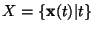andand let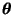denote all the unknown parameters of the model. For notational simplicity, let us denote all the unknown variables by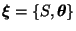. The cost function is then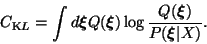(5)

The two things needed for equation 5 are the exact formulation of the posterior density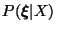and its parametric approximation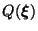.

According to the Bayes' rule, the posterior pdf of the unknown variables S andis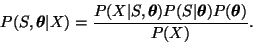The term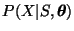is obtained from equations 2-4; the distribution of the data is the same as for the noise n(t) except that its mean is corrected by the prediction given by the model. Let us denote the vector of the means of n(t) by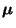and the vector of the variances by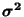. The distribution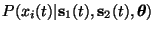is thus Gaussian with mean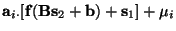and variance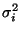. Here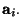denotes the ith row vector of A. As usually, the noise components ni(t) are assumed to be independent and therefore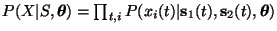.

The terms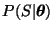and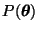are also products of simple Gaussian distributions and they are obtained directly from the definition of the model structure. The term P(X)is not a function of any of the parameters of the model and can be neglected.

The approximation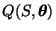needs to be simple for mathematical tractability and computational efficiency. We assume that it is Gaussian density with a diagonal covariance matrix. This means that the approximation is a product of the independent distributions: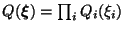. The parameters of each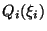are the mean and variance which will be denoted by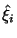and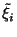, respectively.

Both the posterior density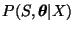and its approximationare products of simple Gaussian terms, which simplifies the cost function considerably: it splits into expectations of many simple terms. The terms of the form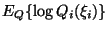are the negative entropies for Gaussians and have the values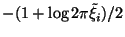. The most difficult terms are of the form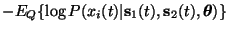. They are approximated by applying the second order Taylor's series expansions of the nonlinear activation functions as explained in . The rest of the terms are expectations of simple Gaussian terms, whose expectations can be computed as in .

The cost function CKL is a function ofand, i.e., the posterior means and variances of the latent variables and the parameters of the network. This is because instead of finding a point estimate, a whole distribution will be estimated for the latent variables and the parameters during learning.Next: Simulations Up: Multi-Layer Perceptrons as Previous: Model structure
Harri Lappalainen
1999-05-25